# How to Calculate and Solve for Monochromatic Emissive Power | Radiation Heat Transfer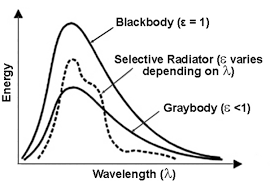The image above represents monochromatic emissive power.

To compute for monochromatic emissive power, five essential parameters are needed and these parameters are Planck’s Constant (h), Velocity of Light (c), Wavelength (λ), Boltzmann’s Constant (KB) and Temperature (T).

The formula for calculating monochromatic emissive power:

ebx = 2πhc²λ-5 / exp(ch/KBλT) – 1

Where:

ebx = Monochromatic Emissive Power | Planck’s Equation
h = Planck’s Constant
c = Velocity of Light
λ = Wavelength
KB = Boltzmann’s Constant
T = Temperature

Let’s solve an example;
Find the monochromatic emissive power when the planck’s constant is 6.626E-24, the velocity of light is 3E8, the wavelength is 22, the boltzmann’s constant is 1.380E-23 and the temperature is 10.

This implies that;

h = Planck’s Constant = 6.626E-24
c = Velocity of Light = 3E8
λ = Wavelength = 22
KB = Boltzmann’s Constant = 1.380E-23
T = Temperature = 10

ebx = 2πhc²λ-5 / exp(ch/KBλT) – 1
ebx = 2π(6.62607004e-34)(300000000)²(22)-5 / exp((300000000)(6.62607004e-34)/(1.38064852e-23)(22)(10)) – 1
ebx = 2π(6.62607004e-34)(90000000000000000)(1.94037e-7) / exp(1.987821012e-25/3.037426744e-21) – 1
ebx = 7.270512005456302e-23 / exp(0.00006544424539379114) – 1
ebx = 7.270512005456302e-23 / 1.0000654463869152 – 1
ebx = 7.270512005456302e-23 / 0.00006544638691519111
ebx = 1.11e-18

Therefore, the monochromatic emissive power is 1.11e-18.

Nickzom Calculator – The Calculator Encyclopedia is capable of calculating the monochromatic emissive power.

To get the answer and workings of the monochromatic emissive power using the Nickzom Calculator – The Calculator Encyclopedia. First, you need to obtain the app.

You can get this app via any of these means:

To get access to the professional version via web, you need to register and subscribe for NGN 2,000 per annum to have utter access to all functionalities.
You can also try the demo version via https://www.nickzom.org/calculator

Apple (Paid) – https://itunes.apple.com/us/app/nickzom-calculator/id1331162702?mt=8
Once, you have obtained the calculator encyclopedia app, proceed to the Calculator Map, then click on Materials and Metallurgical under Engineering.Now, Click on Radiation Heat Transfer under Materials and MetallurgicalNow, Click on Monochromatic Emissive Power under Radiation Heat Transfer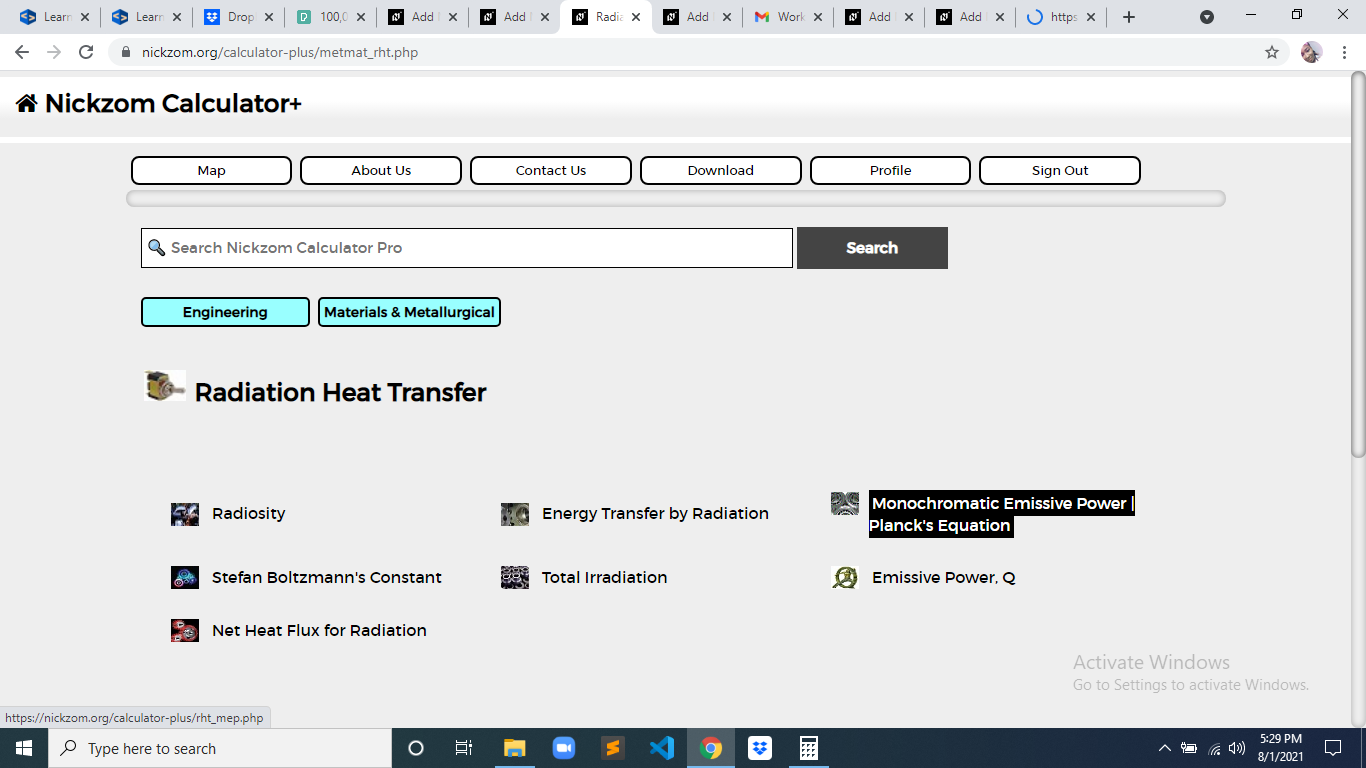The screenshot below displays the page or activity to enter your values, to get the answer for the monochromatic emissive power according to the respective parameter which is the Planck’s Constant (h), Velocity of Light (c), Wavelength (λ), Boltzmann’s Constant (KB) and Temperature (T).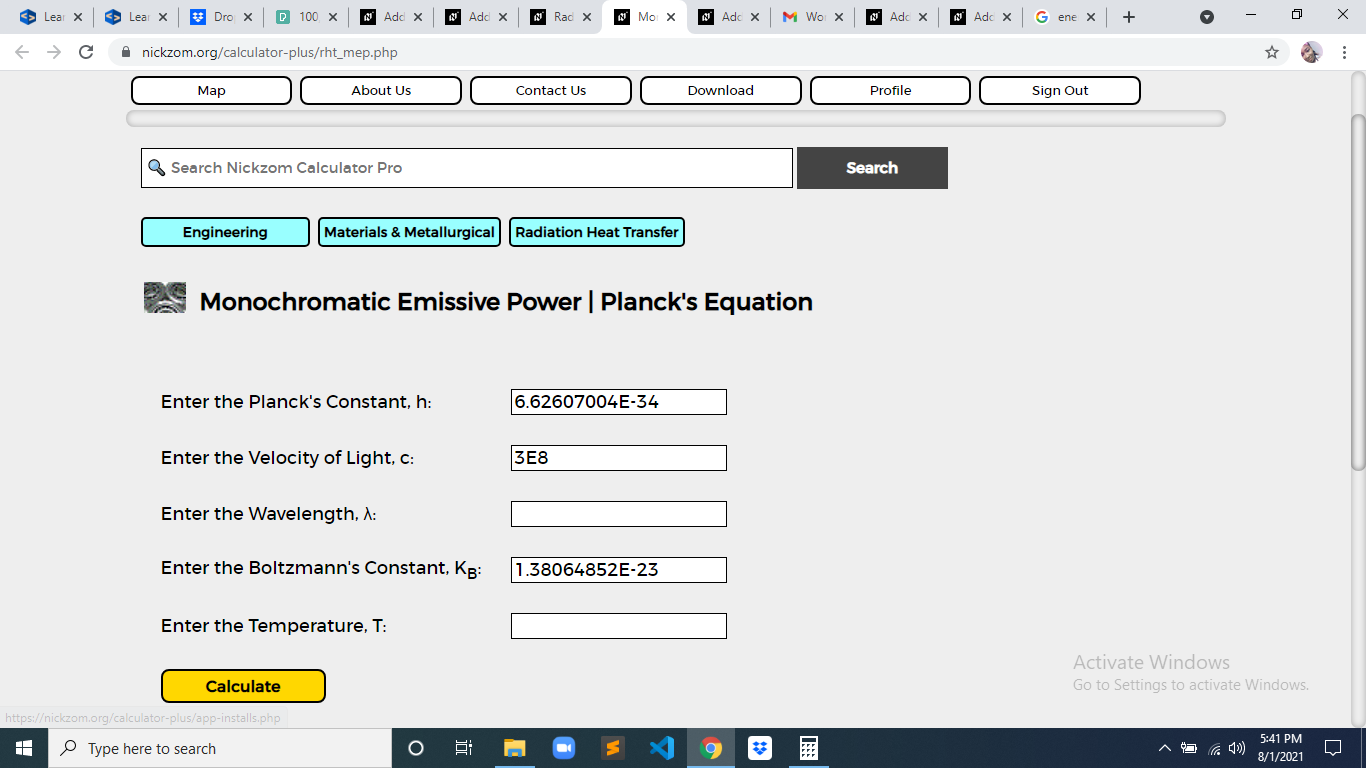Now, enter the values appropriately and accordingly for the parameters as required by the Planck’s Constant (h) is 6.626E-34, Velocity of Light (c) is 3E8, Wavelength (λ) is 22, Boltzmann’s Constant (KBis 1.380E-23 and Temperature (T) is 10.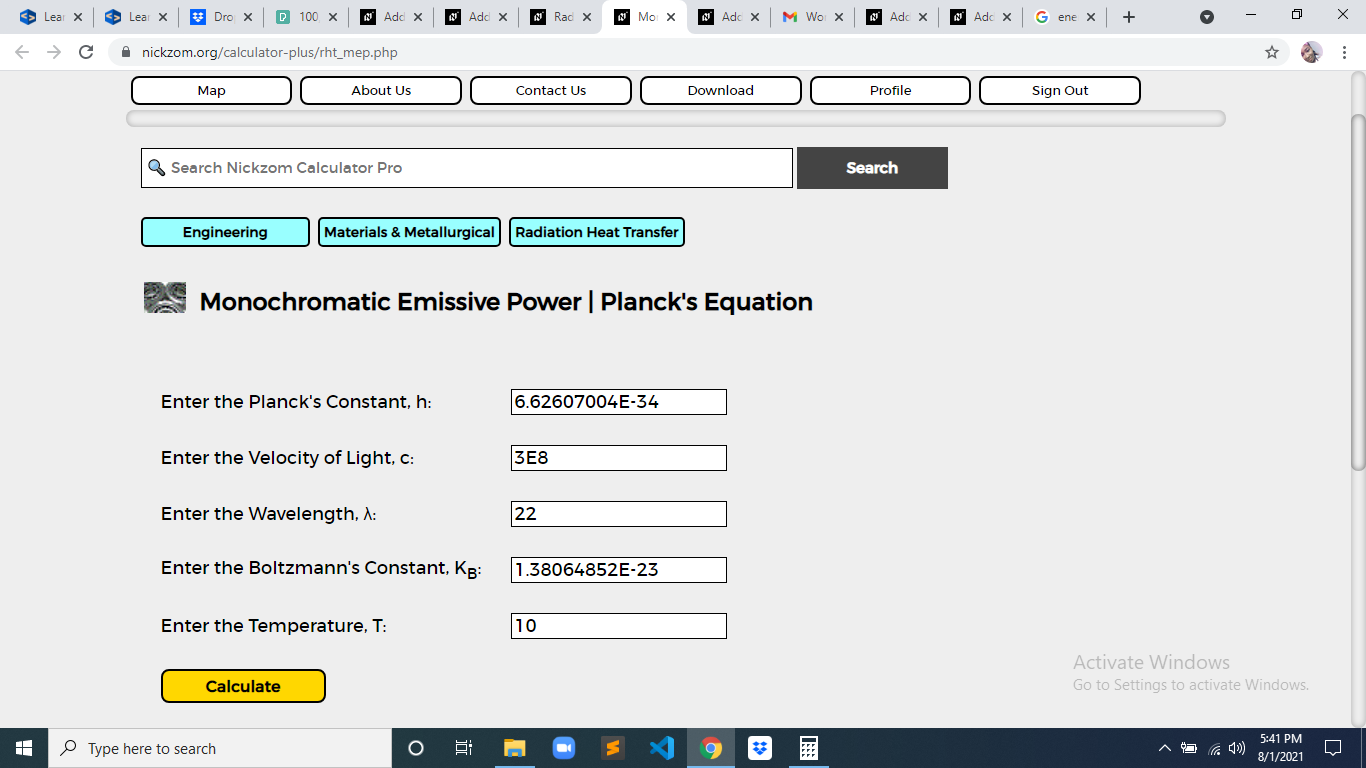Finally, Click on Calculate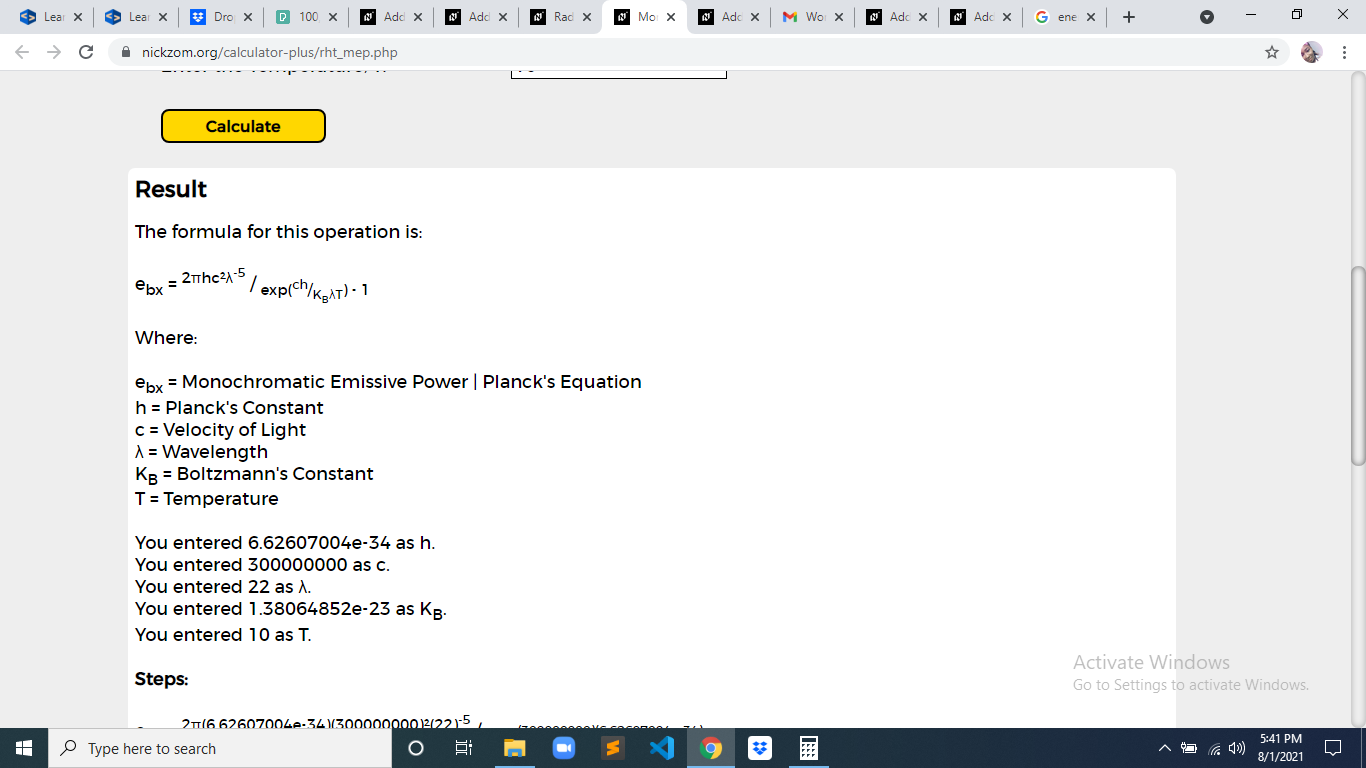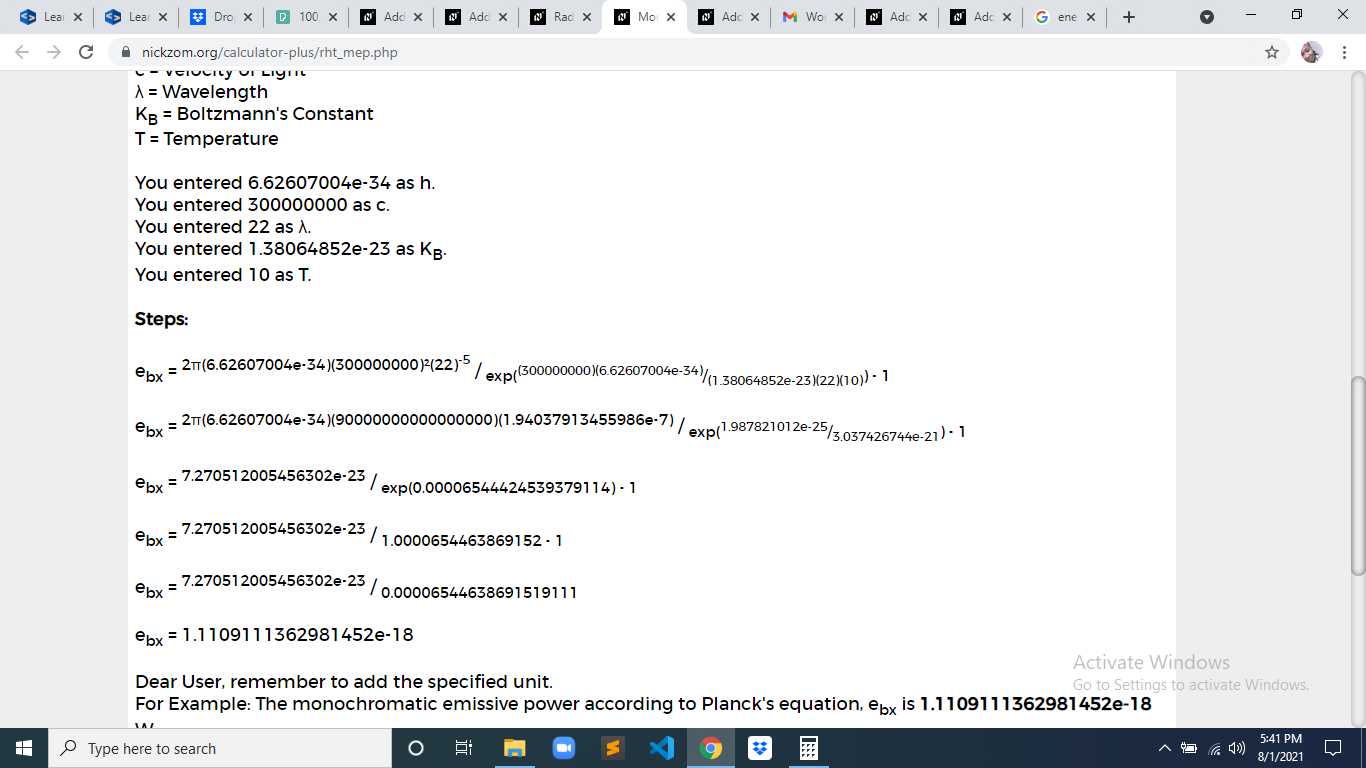As you can see from the screenshot above, Nickzom Calculator– The Calculator Encyclopedia solves for the monochromatic emissive power and presents the formula, workings and steps too.